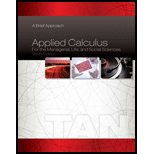Chapter 5.5, Problem 22E### Applied Calculus for the Manageria...

10th Edition
Soo T. Tan
ISBN: 9781285464640

#### Solutions

Chapter
Section### Applied Calculus for the Manageria...

10th Edition
Soo T. Tan
ISBN: 9781285464640
Textbook Problem

# find the derivative of the function.22 f ( x ) = ln x + x

To determine

To find: The derivative of the given function.

Explanation

Given:

The given function is,

f(x)=lnx+x=(lnx+x)1/2 (1)

Formula used:

The chain rule for the derivative of the function f(x)=g(t(x)) with respect to x, where t(x)=u is,

ddx(f(x))=ddu(g(u))ddx(t(x))

Calculation:

Use chain rule to differentiate both side of the equation (1) with respect to x.

ddxf(x)=ddx(lnx+x)1/2f'(x)=ddu(u)1/2ddx(lnx+x)=12u(121)(1x

### Still sussing out bartleby?

Check out a sample textbook solution.

See a sample solution

#### The Solution to Your Study Problems

Bartleby provides explanations to thousands of textbook problems written by our experts, many with advanced degrees!

Get Started

## Additional Math Solutions

#### Find more solutions based on key concepts